## Video Game -- Particle Beam

Introduction.

You will produce a game in which ball-like particles enter from one side of the screen. You must use a paddle to bounce the balls into collectors to get points. The paddle position will be controlled by an analog input. There will be a time limit to the game. Display will be on an 320x240 TFT LCD, with DMA sound effects. The balls will follow standard billards-type dynamics, with zero friction between balls. An example of billard dynamics is shown below. The sound must be produced through a DAC output using a DMA channel.

Procedure:

In this lab and every lab, make sure you are running Protothreads 1_2_2 or later.
There is a fixed point animation example on the Dev Board page, including forces for gravity and drag (video).
All TFT connections from lab 2 stay the same and are wired for you on the big board.
Most of the DAC connections stay the same, except as noted below.

Sound effects using DMA SPI:
The SPI DAC you will use is the MCP4822 as in Lab 2. But you will be using DMA to drive the SPI channel, so you need to modify the chip-select pin on SPI2 to use framed SPI. This mode allows the SPI controller to toggle the chip select line, implying that only one peripherial can be attached to that channel, but simplfying program logic. See section 23.3.6 in the reference manual. Specifically, this feature allows a DMA channel to blast data out to a DAC or other fast device without using an ISR. Get sound output by connecting the DAC output (DACA or DACB) to the speakers.
DMA setup:
-- The logic might be to set up a DMA channel hardware-triggered by a timer event, and possibly using auto-repeat.
See the first example on the SPI page for details about framed SPI, but note that this example, while framed, does not use DMA.
-- Use a DMA memory-source (flash or RAM) corresponding to a table of voltages, or-ed with the DAC control word in the high-bits.
-- Use as a DMA memory-destination the SPI transmit register.
-- The DMA could be operated one-shot for a short sound effect, or in auto-repeat mode for a longer signal.
The length of the longer signal could be based on a timed thread event.

ADC input AN11 pin 24 using the potentiometer circuit shown below.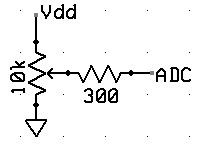The game will be controlled by a trimpot potentiometer hooked to an ADC input on the PIC32. Use the circuit to the left to make a user-variable voltage. The Development Board page shows how to set up the A/D converter to read a voltage in a thread. The example reads the AN11 analog input and draws the voltage on the TFT.

Trimpot schematic: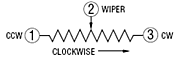bottom view: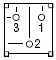image: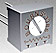Fixed Point arithmetic

Floating point is too slow for animation, so you will be using a fixed point data type and doing all arithmetic in fixed point.
The animation example linked above uses the _Accum data type.

Dynamics:

You are going to be programming in the equations of motion for the balls. Remember that the video coordinate system has x increasing to the right and y increasing downward. We will step the billards system forward in time by calculating the total change in velocity from a collision, without worrying exactly how forces change the velocity.

The change in velocity during impact can be derived for frictionless balls of equal mass by noting that the the impact force must act in a direction parallel to the line connecting the centers of the two impacting balls. The change in velocity must be parallel to the connecting line also, with the velocity component parallel to the line having its sign reversed by the collision and the velocity component perpendicular to the line unchanged. Projecting the initial velocity onto the line connecting the centers, negating the result, and resolving it back into x and y velocity components gives the velocity change. If i and j are the indices of the colliding balls, define: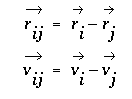then delta v for ball i is given by the following where the right-most term represents the projection of the velocity onto the line and the other term converts the projection back to x,y coordinates.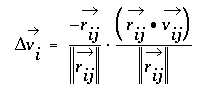The calculation procedure for each time step is:

1. Compute the Δv for each collision, based on the positions of the balls, and add it to the current velocity. Do this for every pair of balls. This step is time consuming, but only has to be performed for any two balls if they are less than 2 radii apart. Ball-ball collisions will not be exact because of finite time steps. One consequence of this is that balls tend to capture each other when they collide. You will need to do a numerical hack to avoid capture. I suggest that after a ball collides with another ball or the paddle, that it not be allowed to collide again for a few frames. For collision debugging, it will be useful to slow down particles to less than one pixel/frame.
Pseudocode for this might be:
```For each ball i from 1 to n   For each ball j from i+1 to n     Compute approximate rij by checking:     if Δx and Δy are both less than 4      if (||rij||2 less than (2*(ballRadius))2 and hitCounteri is zero)         Compute vij         Compute Δvi         Add Δvi to vi         Subtract Δvi from vj         Set hitCounteri big enough to avoid particle capture      elseif (hitCounteri>0)         decrement hitCounteri      endif     endif   endfor endfor ```
2. Using _Accum fixed point, any Δx or Δy greater than 181 (sqrt(32767)) will overflow when squared and produce erratic results.
This means that BEFORE you square the deltas, you should do the very quick check to see if both deltas are less than 2 ball radii.
3. When I coded this, I did not bother to calculate the square root of the sum of squares when calculating ||`rij`|| (too slow).
Compare the squares.
4. When dividing by ||`rij`||2 you need to be careful not to divide by zero (causes reset). A low ||`rij`||2 implies a head-on collision,
so if ||`rij`||2 <1, just switch the velocities of the two balls. Otherwise, do the divide, perhaps by table-lookup.
5. For each ball (and after the `Δvi` from any collision is applied for that ball), simulate friction between the ball and table by making
`vx(t+dt)=vx(t)-vx*drag` and ```vy(t+dt)=vy-vy*drag ```The drag should be small, perhaps` drag=0.001 `( but converted to fixed notation).
6. Update the positions according to
`x(t+dt)=x(t)+vx*dt` and` y(t+dt)=y(t)+vy*dt`
7. Detect collisions with the walls and modify velocities by negating the velocity component perprendicular to the wall.
8. Delete any balls which are collected, or which make it past the collector to the left side of the screen, and modify the score

Clearly, v and x all need initial conditions, which you will set, according the specifications below. It is doubtful that you will have enough time between frames to do all of the calculations in floating point. I suggest using 32 bit, signed numbers with the binary point set at the 16-bit boundary. I also suggest scaling velocity so that you can make dt=1, thereby avoiding a multiply. You can think of this as: (1) Units of distance are PIXELS, (2) Units of velocity are PIXELS/FRAME. As examples, any of the NTSC particle systems are done with fixed point numbers.

The previous analysis is adapted from: Studies in Molecular Dynamics. I. General Method, by B. Alder and T. Wainwright,
Journal of Chemical Physics, Vol 31 #2, Aug 1959, pp 459-466. See also Hard-Sphere molecular dynamics.

Results:
2016: video On TFT

Week one required checkpoint

By the end of lab session in week one of the lab you must have TWO of the following:

• One ball bouncing correctly inside the playing field, as defined below.
• DMA produced sound
• ADC controlling the paddle on screen including the playing field image.
• Finishing a checkpoint does NOT mean you can leave lab early!

Week two required checkpoint

By the end of lab session in week two of the lab you must have ALL of the following:

• DMA produced sound.
• ADC controlling the paddle on-screen and including the playing field image.
• Balls interacting correctly with the paddle and all playing field surfaces.
• Two balls bouncing off each other correctly inside the playing field.
• Finishing a checkpoint does NOT mean you can leave lab early!

Week three Assignment

Write a program in C using ProtoThreads with these specifications:

• When you press the game start button (not reset), the program should:
• draw a playing field consisting of a rectangle 320 wide x 240 high on the LCD screen with two internal barriers.
The barriers should be placed at about 1/3 the x-width of the screen and and about 1/4 of the screen long.
Balls bounce off the sides of the barriers.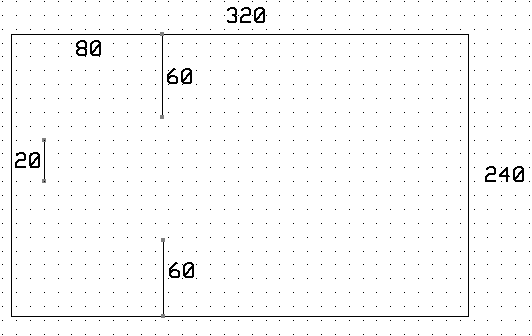• set the running time clock to zero. The clock should read elapsed play time on secreen.
• start firing balls of radius 2 pixels onto the right hand edge of the screen with vx=-1 to -3 pixels/frame and vy=+1.5 to -1.5 pixels/frame.
The speed distribution in the y-direction must be uniform and random. The x speed can be fixed between the values indicated.
You can vary this to make the game more playable, if necessary. The ball icons can be very simple.
• draw a "paddle" consisting of a line segment with a size about 5 times the size of the balls. You can vary this to make the game more playable, or to produce harder levels of play.
• Set the TFT frame time using a thread to be faster than 15/second.
Since the computation will be the most demanding calculation and depends on the number of balls,
arrange the thread to produce a constant frame rate, while allowing as much time as possible for computation.
(pseudocode example of constant frame rate)
Display the frame rate on the screen in frames/sec.
• At each frame time, update the velocity and position of all the balls on the screen, and redraw the paddle.
The drawing of the paddle need not be complicated.
• Balls which are hit on the front of the catcher increment your score,, and the balls are removed from the screen.
• Balls which get past the catcher to the left side of the screen decrement your score, and the balls are removed from the screen.
• A score, a frame rate, number of current balls, and time should be displayed.
• All balls can be deflected by other balls according to the hard ball dynamics given above.
• Paddle vertical position on the screen should be changed by a potientiometer attached to the A/D converter.
The paddle horizontal position will be fixed at around x=20.
• The sides of the barriers, and the walls reflect balls according to: angle incidence equals angle of reflection.
• The game ends after a fixed time, which you can choose.
• New balls should enter the playing field at regular intervals (from the right hand edge), perhaps a few per second.
Balls which leave the screen are immediately recycled to to sent in again.
• You will be graded on the number of simultaneous balls you can animate. You must animate at least 30 balls.
You will need to optmize your code and use fixed point.
• There should be minimal visual artifacts (tearing, flickering) during operation.
• There are required sound effects, which for full credit, must be generated using DMA-driven, SPI DAC output, with NO sound-generation ISR.
Audio synthesis rate should b 44 Ksamples/sec for at least one sound. Others can be at lower rate.
You need a sound for +1 score, -1 score, and for game end.

When you demonstrate the program to a staff member, you should play the game.
At no time during the demo should you need to press RESET.

Your written lab report should include the sections mentioned in the policy page, and :

• The DMA setup for Vref output.
• The details of your integration algorithm (Euler? Verlet?, Full hard disc?).
• An image of the game from the TFT LCD.
• A heavily commented listing of your code.